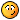# ExcelExcel 2007 Formula Help Needed

#### scyllanbay

I have one formula that is looking in a column range (Z:Z) and counting a specific date period (1/29/2012 thru 2/1/2013).

=COUNTIF(\$Z\$3:\$Z\$1063,">=1/29/2012")-COUNTIF(\$Z\$3:\$Z\$1063,">=2/1/2013")

I have another forumula that is looking in a different column (AR:AR) and counting the number of cells that contain a number less than or equal to 0.

=COUNTIF(AR:AR,"<=0")

I now need a formula that will say....within that first range of dates (1/29/2012 thru 2/1/2013)...how many of those dates contain a number less than or equal to 0 in column AR.

How would I go about doing this?#### alow

In Excel 2007, you can use the COUNTIFS formula. I think this would work for you:

=COUNTIFS(\$Z\$3:\$Z\$1063,">=1/29/2012",\$Z\$3:\$Z\$1063,"<=2/1/2013",\$AR\$3:\$AR\$1063,"<=0")

You have to adjust the ranges so they are the same, but I think that will do it. Also, you can use the first 4 arguments of that to replace your subtraction for counting cells in that date range if you wanted to simplify that.